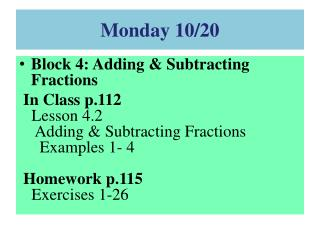# Monday 10/20 - PowerPoint PPT PresentationDownload PresentationMonday 10/20

Monday 10/20
Download Presentation## Monday 10/20

- - - - - - - - - - - - - - - - - - - - - - - - - - - E N D - - - - - - - - - - - - - - - - - - - - - - - - - - -
##### Presentation Transcript

1. Monday 10/20 • Block 4: Adding & Subtracting Fractions In Class p.112Lesson 4.2Adding & Subtracting Fractions Examples 1- 4Homework p.115Exercises 1-26

2. Warm-Up 8 • What is the least common denominator for ? • What is the least common denominator for ? Replace the ? with a number to make the two fractions equivalent. 3. 4. 15 8 9

3. Lesson 4.2 Adding and Subtracting Fractions Find sums and differences of fraction expressions.

4. Add or Subtract Fractions With Common Denominators • Add or subtract the numerators. • Write the sum or difference over the common denominator. • Simplify the fraction.

5. Add or Subtract Fractions With Unlike Denominators • Rewrite the fractions using the least common denominator (LCD). • Add or subtract the numerators. • Write the sum or difference over the common denominator. • Simplify the fraction.

6. Example 1 Kiley practiced of her piano music in the morning. That afternoon she practiced of her music. What fraction of her music did she practice? Write the problem. Add the numerators and write the sum over the common denominator. Simplify the fraction. Kiley practiced of her piano music.

7. Example 2 Find the value of . Subtract the numerators. Write the difference over the common denominator. Simplify the fraction.

8. Example 3 Mallory made gallon of ice cream. Logan made gallon. How many total gallons of ice cream do they have together? Write the problem. Find the least common denominator for the set of fractions. Rewrite the fractions using the LCD.

9. Example 3 Continued… Mallory made gallon of ice cream. Logan made gallon. How many total gallons of ice cream do they have together? Add the numerators. Change the improper fraction to a mixed number. Mallory and Logan made a total of gallons of ice cream.

10. Example 4 Find the value of . Find the LCD. 3: 3, 6, 9, 12 4: 4, 8, 12, 16 Rewrite fractions using using the LCD. Subtract the numerators.

11. Monday 10/20 • Block 4: Adding & Subtracting Fractions In Class p.112Lesson 4.2Adding & Subtracting Fractions Examples 1- 4Homework p.115Exercises 1-26

12. Exit Problems Find the value of:

13. Explore! Pizza Party! Janice was ordering pizzas for her friends. The table shows the fractions of the pizzas each person said they could eat. How much cheese pizza do Lakelynn and Alvaro think they can eat together? Lay out the fraction tiles to represent the amounts of cheese pizza which Lakelynn and Alvaro want. Combine the fraction tiles to find the total. Simplify the fraction, if needed. Draw a picture of the fraction model you used and write the addition equation on paper.

14. Explore! Pizza Party! Step 1 Follow the process shown for Lakelynn and Alvaro to answer the following questions. a. How much cheese pizza will Janice and Jory eat together? b. How much Hawaiian pizza will Jory and Lakelynn eat together? c. How much pepperoni pizza will Lakelynn and Alvaro eat together? Step 2Look at the equations. What do you notice about the denominators of the fractions being added and the denominator of the answer? What about the numerators? How might you add fractions with common denominators without using fraction tiles?

15. Explore! Pizza Party! How much Hawaiian pizza with Jory and Alvaro eat? Lay out fraction tiles to represent the amount of Hawaiian pizza Jory and Alvaro eat. Find the least common denominator of the two fractions. 2: 2, 4, 6, 8 3: 3, 6, 9, 12 LCD = 6 Replace the original fraction tiles with equivalent fractions using the LCD. Combine the tiles to find the answer.

16. Explore! Pizza Party! Simplify the fraction, if needed. All improper fractions should be written as mixed numbers. Step 3Follow the process shown for Jory and Alvaro to answer the following questions. Draw pictures of the fraction tiles used in the process. Write the addition equation on your paper. a. How much cheese pizza will Janice and Lakelynn eat? b. How much Hawaiian pizza will Lakelynn and Alvaro eat? c. How much Hawaiian pizza will Janice and Jory eat? d. How much pepperoni pizza will Janice and Alvaro eat? e. How much pepperoni pizza will Janice and Jory eat? f. How much pepperoni pizza will Alvaro and Jory eat? Step 4How might you add fractions with unlike denominators without using tiles?

17. Communication Prompt Is it easy for you to use fraction tiles when adding or subtracting fractions? Why or why not?